# Pandas – GroupBy One Column and Get Mean, Min, and Max values

We can use Groupby function to split dataframe into groups and apply different operations on it. One of them is Aggregation. Aggregation i.e. computing statistical parameters for each group created example – mean, min, max, or sums.

Let’s have a look at how we can group a dataframe by one column and get their mean, min, and max values.

Example 1:

 `import` `pandas as pd ` ` `  ` `  `# creating a dataframe ` `df ``=` `pd.DataFrame([(``'Bike'``, ``'Kawasaki'``, ``186``), ` `                   ``(``'Bike'``, ``'Ducati Panigale'``, ``202``), ` `                   ``(``'Car'``, ``'Bugatti Chiron'``, ``304``),  ` `                   ``(``'Car'``, ``'Jaguar XJ220'``, ``210``), ` `                   ``(``'Bike'``, ``'Lightning LS-218'``, ``218``),  ` `                   ``(``'Car'``, ``'Hennessey Venom GT'``, ``270``), ` `                   ``(``'Bike'``, ``'BMW S1000RR'``, ``188``)], ` `                  ``columns ``=``(``'Type'``, ``'Name'``, ``'top_speed(mph)'``)) ` ` `  `df `

Output :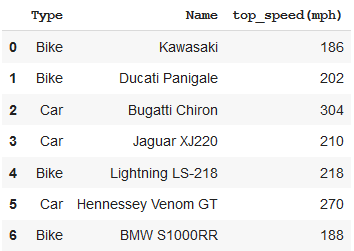Finding mean, min and max values.

 `# using groupby function with aggregation ` `# to get mean, min and max values ` `result ``=` `df.groupby(``'Type'``).agg({``'top_speed(mph)'``: [``'mean'``, ``'min'``, ``'max'``]}) ` ` `  `print``(``"Mean, min, and max values of Top Speed grouped by Vehicle Type"``) ` `print``(result) `

Output :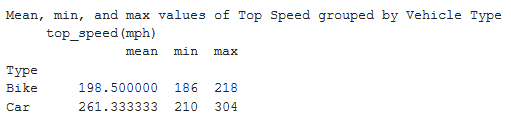Example 2:

 `import` `pandas as pd ` ` `  ` `  `# creating a dataframe ` `sales_data ``=` `pd.DataFrame({ ` `'customer_id'``:[``3005``, ``3001``, ``3002``, ``3009``, ``3005``, ``3007``, ` `               ``3002``, ``3004``, ``3009``, ``3008``, ``3003``, ``3002``], ` `     `  `'salesman_id'``: [``102``, ``105``, ``101``, ``103``, ``102``, ``101``, ``101``, ` `                ``106``, ``103``, ``102``, ``107``, ``101``], ` ` `  `'purchase_amt'``:[``1500``, ``2700``, ``1525``, ``1100``, ``948``, ``2400``, ` `                ``5700``, ``2000``, ``1280``, ``2500``, ``750``, ``5050``]}) ` ` `  `sales_data `

Output :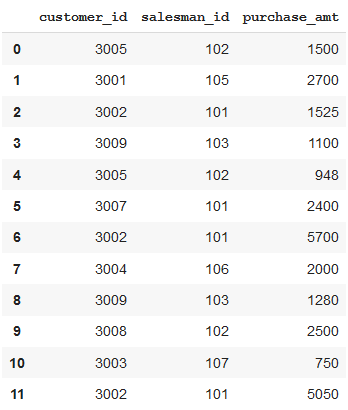Finding mean, min and max values.

 `# using groupby function with aggregation  ` `# to get mean, min and max values ` `result ``=` `sales_data.groupby(``'salesman_id'``).agg({``'purchase_amt'``: [``'mean'``, ``'min'``, ``'max'``]}) ` ` `  `print``(``"Mean, min, and max values of Purchase Amount grouped by Salesman id"``) ` `print``(result) `

Output :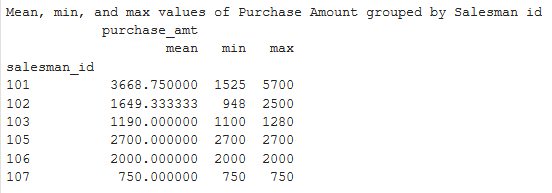Example 3:

 `import` `pandas as pd ` ` `  ` `  `# creating a dataframe ` `df ``=` `pd.DataFrame({``"Team"``: [``"Radisson"``, ``"Radisson"``, ``"Gladiators"``, ` `                            ``"Blues"``, ``"Gladiators"``, ``"Blues"``,  ` `                            ``"Gladiators"``, ``"Gladiators"``, ``"Blues"``,  ` `                            ``"Blues"``, ``"Radisson"``, ``"Radisson"``], ` `                    `  `        ``"Position"``: [``"Player"``, ``"Extras"``, ``"Player"``, ``"Extras"``, ` `                     ``"Extras"``, ``"Player"``, ``"Player"``, ``"Player"``, ` `                     ``"Extras"``, ``"Player"``, ``"Player"``, ``"Extras"``], ` `                    `  `        ``"Age"``: [``22``, ``24``, ``21``, ``29``, ``32``, ``20``, ``21``, ``23``, ``30``, ``26``, ``20``, ``31``]}) ` `df `

Output :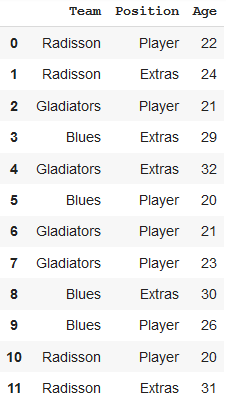Finding mean, min and max values.

 `# using groupby function with aggregation  ` `# to get mean, min and max values ` `result ``=` `df.groupby(``'Team'``).agg({``'Age'``: [``'mean'``, ``'min'``, ``'max'``]}) ` ` `  `print``(``"Mean, min, and max values of Age grouped by Team"``) ` `print``(result) `

Output :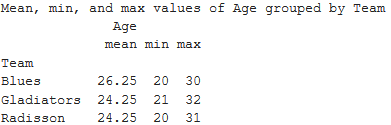My Personal Notes arrow_drop_upCheck out this Author's contributed articles.

If you like GeeksforGeeks and would like to contribute, you can also write an article using contribute.geeksforgeeks.org or mail your article to contribute@geeksforgeeks.org. See your article appearing on the GeeksforGeeks main page and help other Geeks.

Please Improve this article if you find anything incorrect by clicking on the "Improve Article" button below.

Article Tags :

Be the First to upvote.

Please write to us at contribute@geeksforgeeks.org to report any issue with the above content.